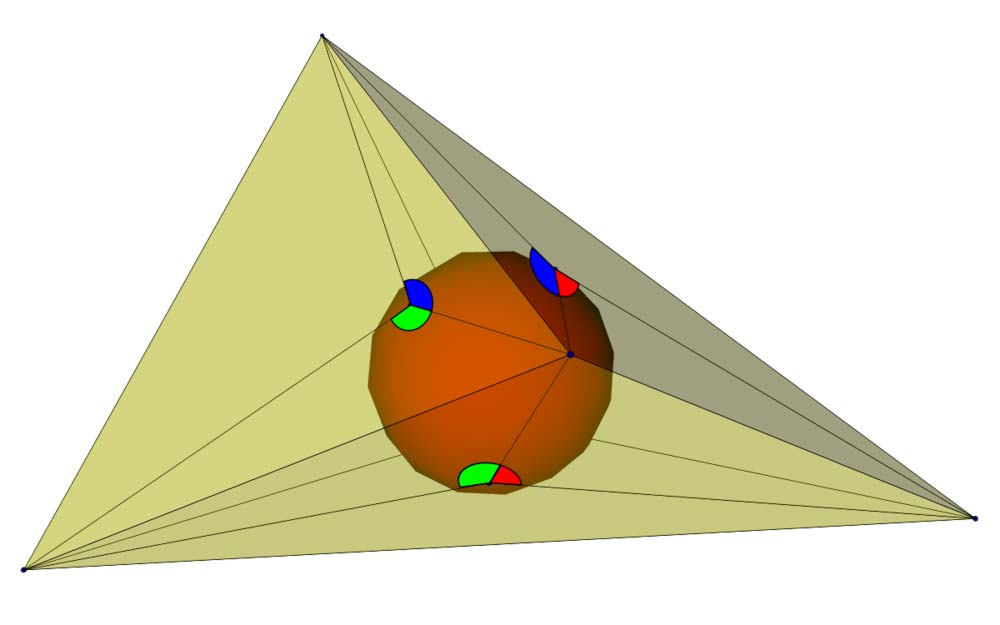A# Bang's theoremBang's theorem states that if all the faces of a tetrahedron have the same perimeter, then the faces are all congruent triangles. This is equivalent to the statement that lines drawn to the vertices of a face of a tetrahedron from the point of contact of the face with the insphere form three angles at the point of contact which are the same three angles in each face. The theorem is named after A. S. Bang, who posed it as a problem in 1897.